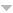# Library

Author TitleSz.-Nagy B., Foias C. Analyse Harmonique des Operateurs de L'Espace de Hilbert
Herz C.S. Analyse harmonique a plusieurs variables.
Levy P. Analyse fonctionnelle.
Levy P. Analyse fonctionnelle.
Fourier M. Analyse Des Equations Déterminées. .
Leroy C.F.A. Analyse Appliquée a la Géométrie des trois dimensions, comprenant les surfaces du second degré. Avec la théorie générale des surfaces courbes et des lignes a double courbure.
Blickensdorfer-Ehlers, Eschmann W.G., Neuzert H., Schelkes K. Analyse 1. Ein Lehr-und Arbeitsbuch fur Atudienanfanger
Ghika A. Analiza functionala.
Salmon G. Analitische Geometrie der Kegelschnitte mit Besonderer Berucksichtigung der neueren methoden
Salmon G. Analitische Geometrie der Hoheren ebenen Curven
Goto M. An Introduction to Topological Groups. Lectures 1973/1974.
Yule G. U. and Kendall M.G. An introduction to the theory of statistics
Davenport W.B., Root W.L. An introduction to the theory of random signals and noise.
Niven I., Zuckerman H.S. An Introduction to the Theory of Numbers. 2nd edit.
Bromwich T.J. I'A An introduction to the theory of infinite series
Turnbull H.W. and Aitken A.C. An introduction to the theory of canonical matrices
Turnbull H.W., Aitken A.C. An Introduction to the Theory of Canonical Matrices
Bocher Maxime An introduction to the study of integral equations
Shaw F.S. An introduction to the relaxation methods
Schwatt I.J. An Introduction to the Operations With Series. Second edition
Melluish R.K. An introduction to the mathematics of map projections.
Cohen A. An Introduction to the Lie Theory of One-Paeameter Groups.With Application of Differential Equations. 2nd edit.
Bartlett M.S.,F.R.S. An Introduction to Stochastic Processes with Special Reference to Methods and Applications
Feller W. An introduction to probability theory and its applications. Vol. I.
Feller W. An introduction to probability theory and its applications. Vol. I.
Bremaud P. An Introduction to Probabilistic Modeling
Houstoun R.A. An introduction to mathematical physics.
Murray F.J. An introduction to Linear Transformation in Hilbert Space
Hilton P.J. An introduction to homotopy theory.
Katznelson Y. An introduction to harmonic nalysis
Katznelson Y. An Introduction to Harmonic Analysis. 2nd edit.corrected
Arrowsmith D.K., Place C.M. An Introduction to Dynamical Systems
Cassels J.W.S. An introduction to diophantine approximation.
Eisenhart L.P. An introduction to differential geometry with use of the tensor calculus.
Brondstred Arne An introduction to convex polytopes
Hoermander L. An Introduction to Complex Analysis in Several Variables.
Subba R.T., Gabr M.M. An Introduction to Bispectral Analysis and Bilinear Time Series Models
Dadourian H.M. An introduction to analytic geometry and the calculus.
Loomis L.H. An introduction to abstract harmonic analysis.
NULL An International Conference on Operator Theory: advances and applications. Honoring I.Gohberg on the occasion of his 60th birthday. Program & abstracts. Univ. Calgary, Algebra, Canada. August 22nd-16th, 1988.